# RS Aggarwal Solutions for Class 9 Chapter 15: Volume and Surface Area of Solids Exercise 15D

## RS Aggarwal Solutions for Class 9 Maths Exercise 15D PDF

The solutions are created by experts with the aim of boosting exam preparation of students. Our subject experts having greater experience in the education industry have designed the solutions based on CBSE guidelines. The answers for the exercise wise problems acts as a reference guide for students to score well in exam. The solutions to all the exercises prepared by our experts are available in PDF format which can be downloaded for free by students. RS Aggarwal Solutions for Class 9 Maths Chapter 15 Volume and Surface Area of Solids Exercise 15D are provided here.

## RS Aggarwal Solutions for Class 9 Chapter 15: Volume and Surface Area of Solids Exercise 15D Download PDF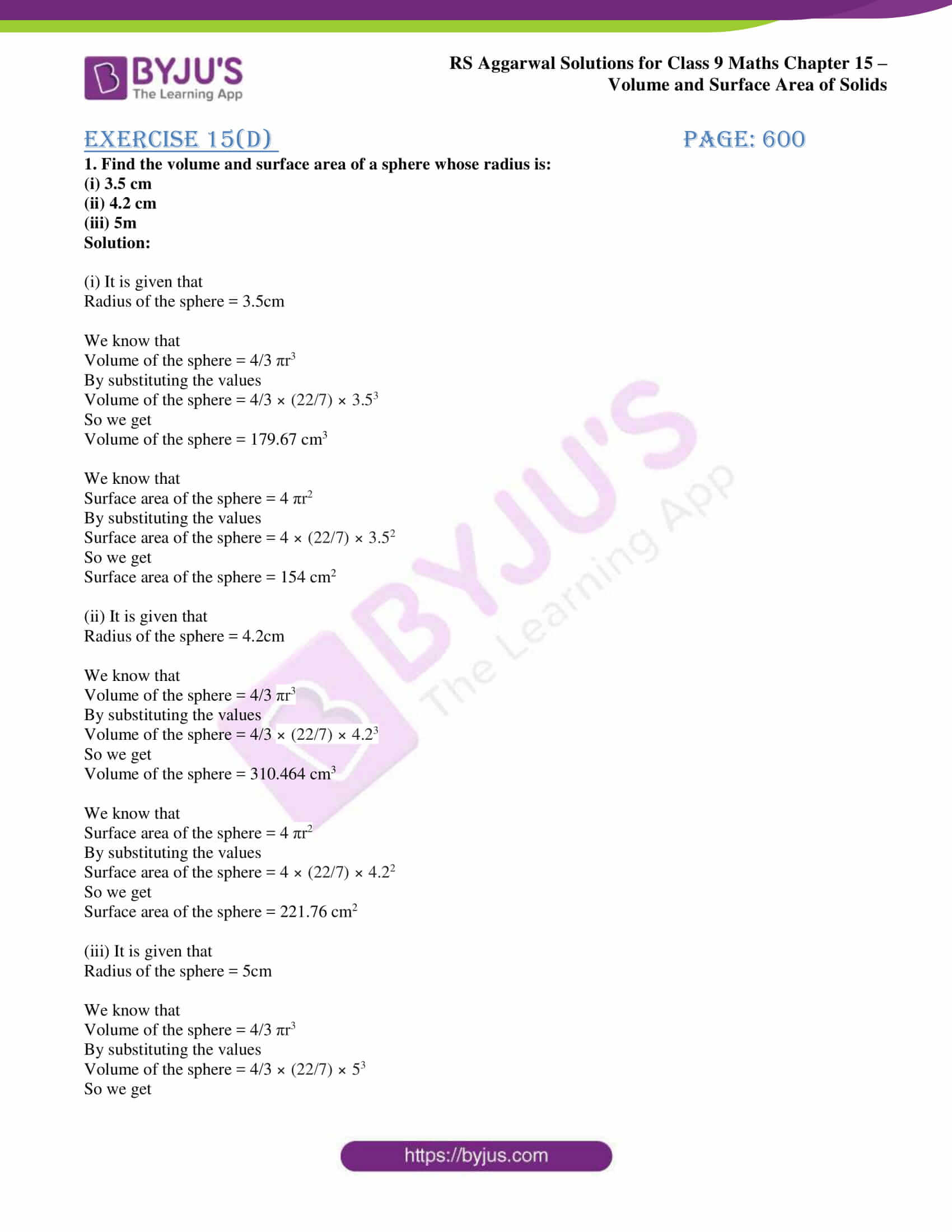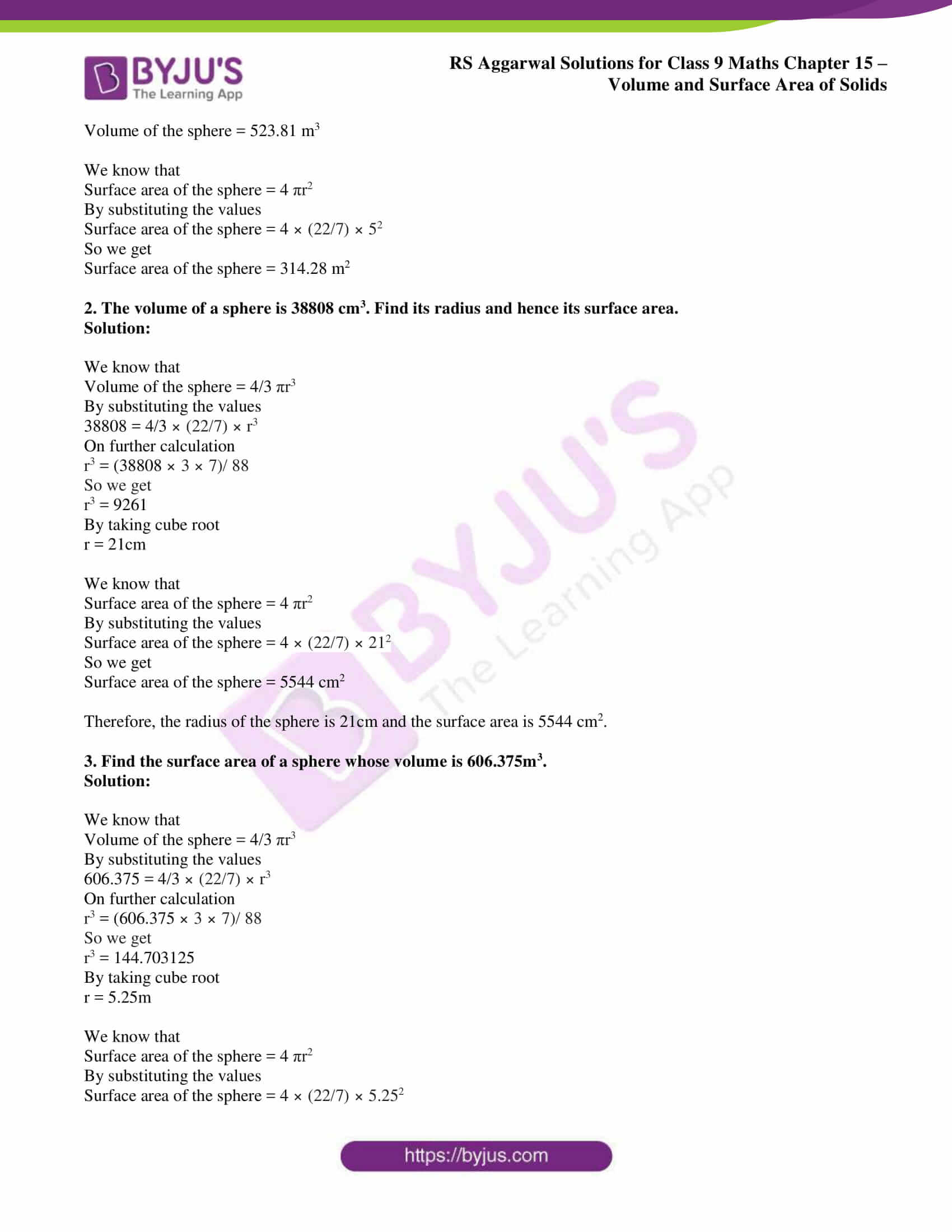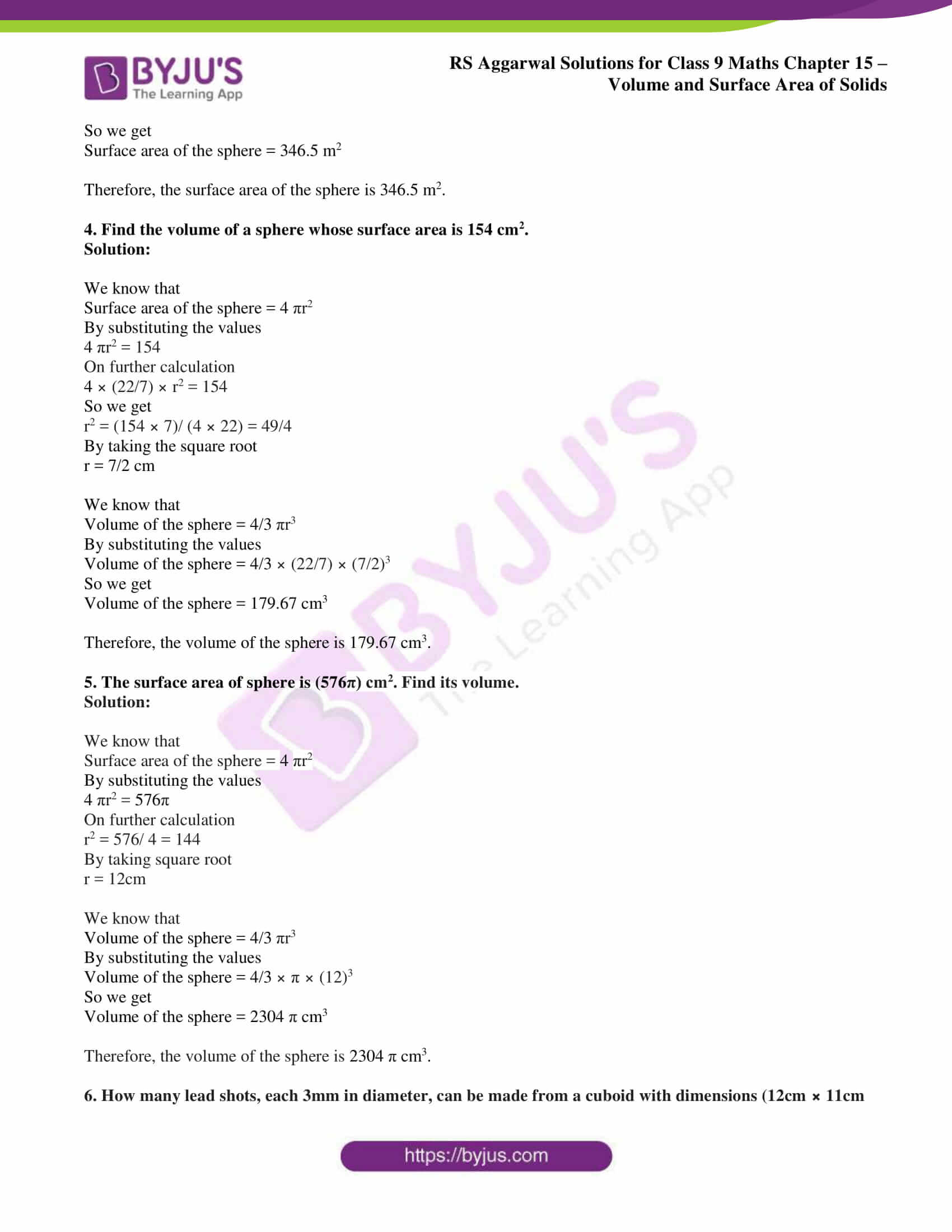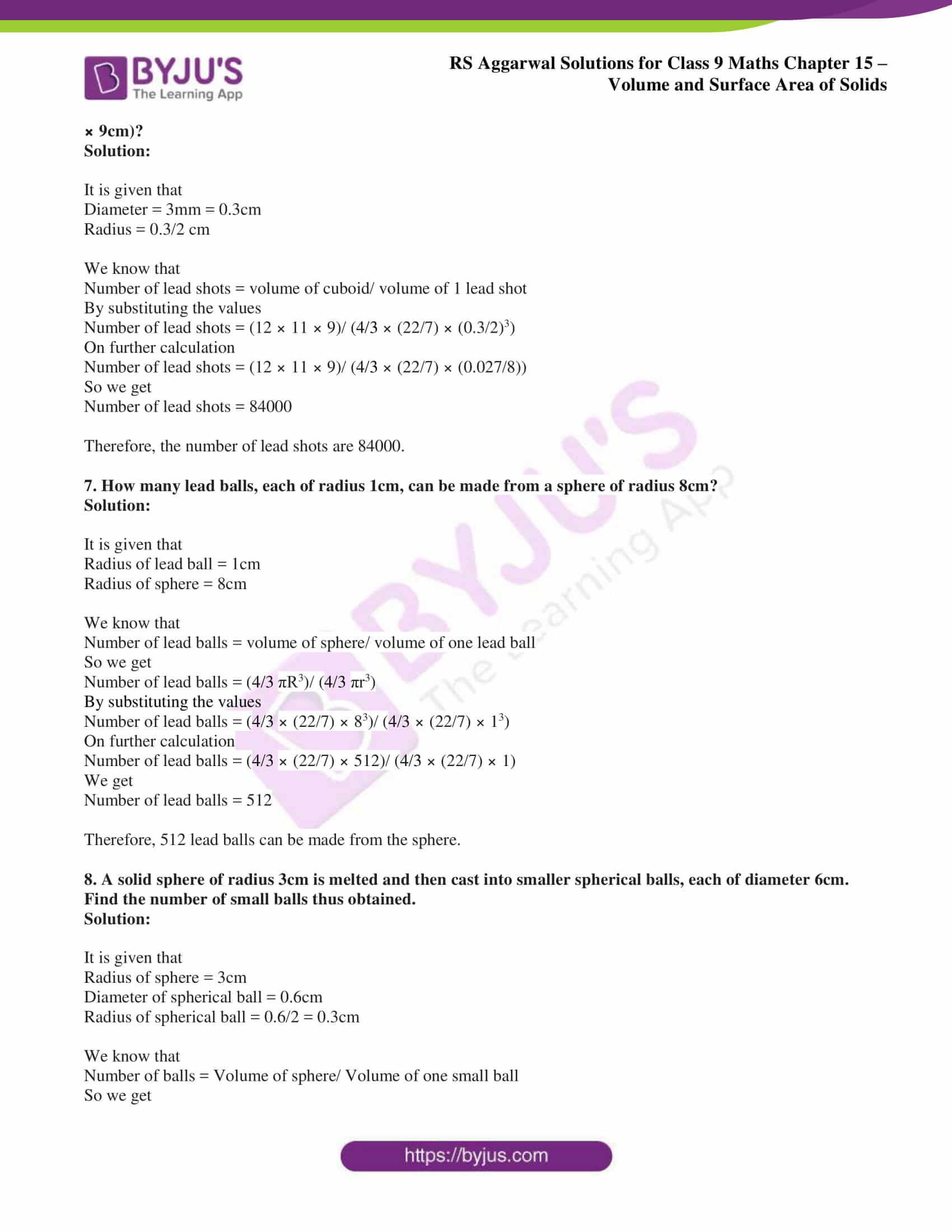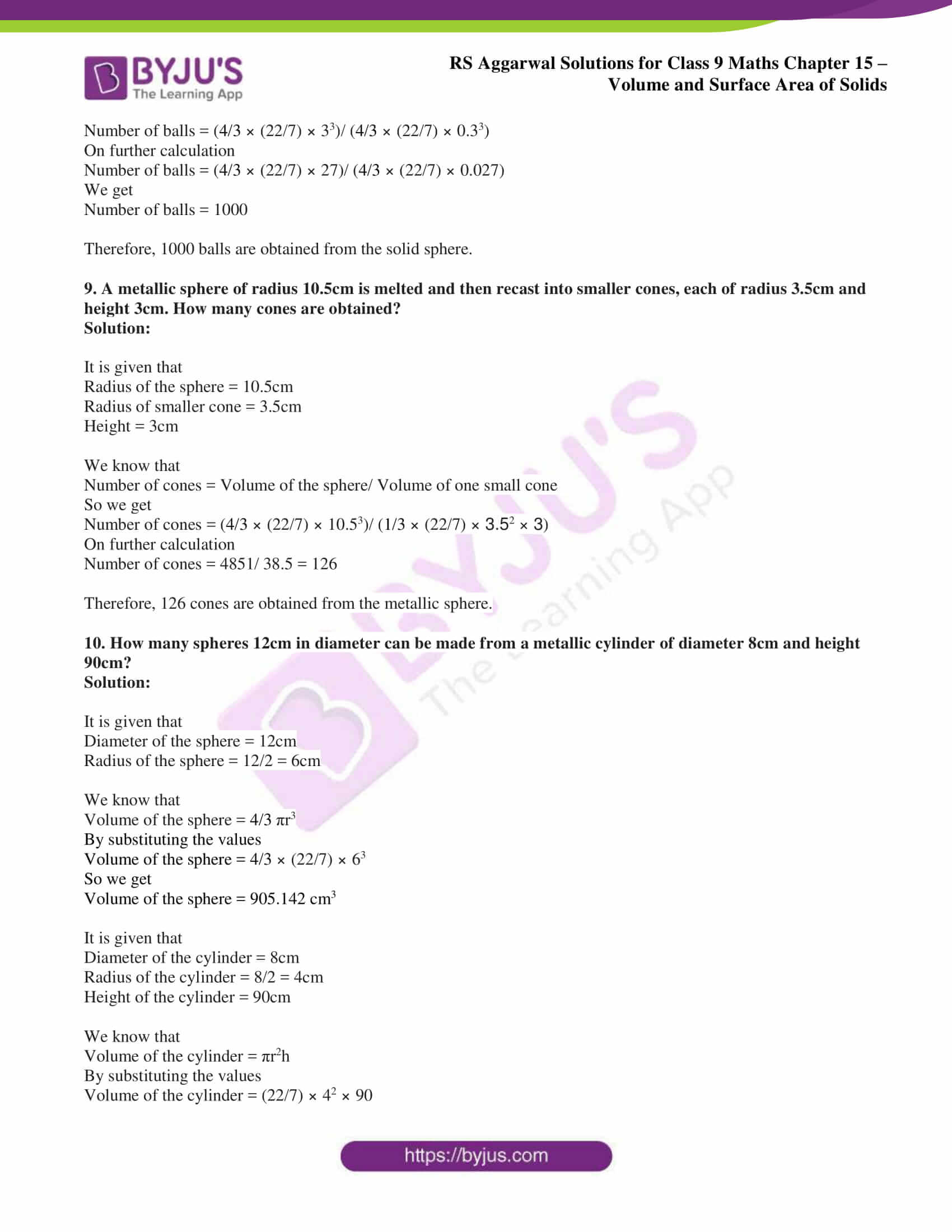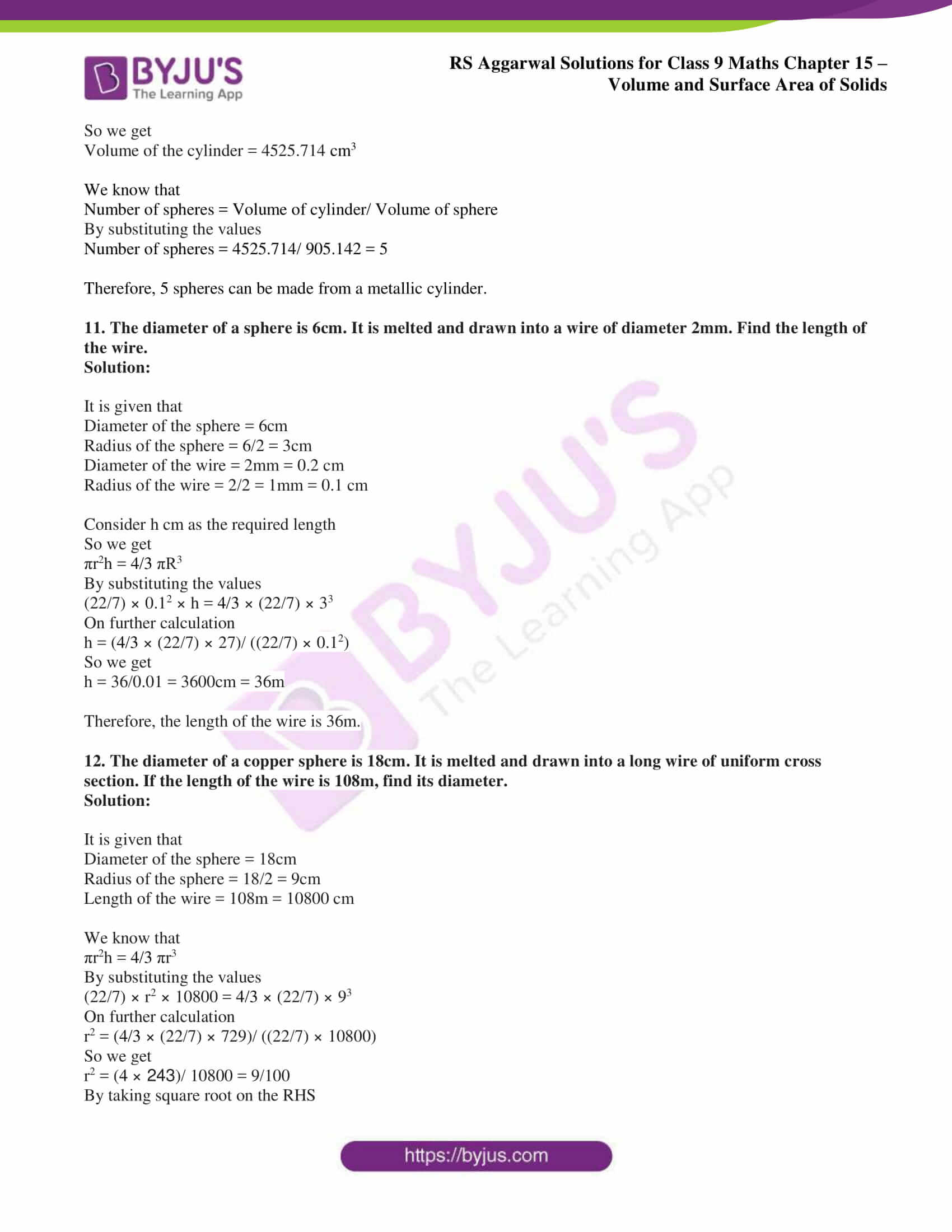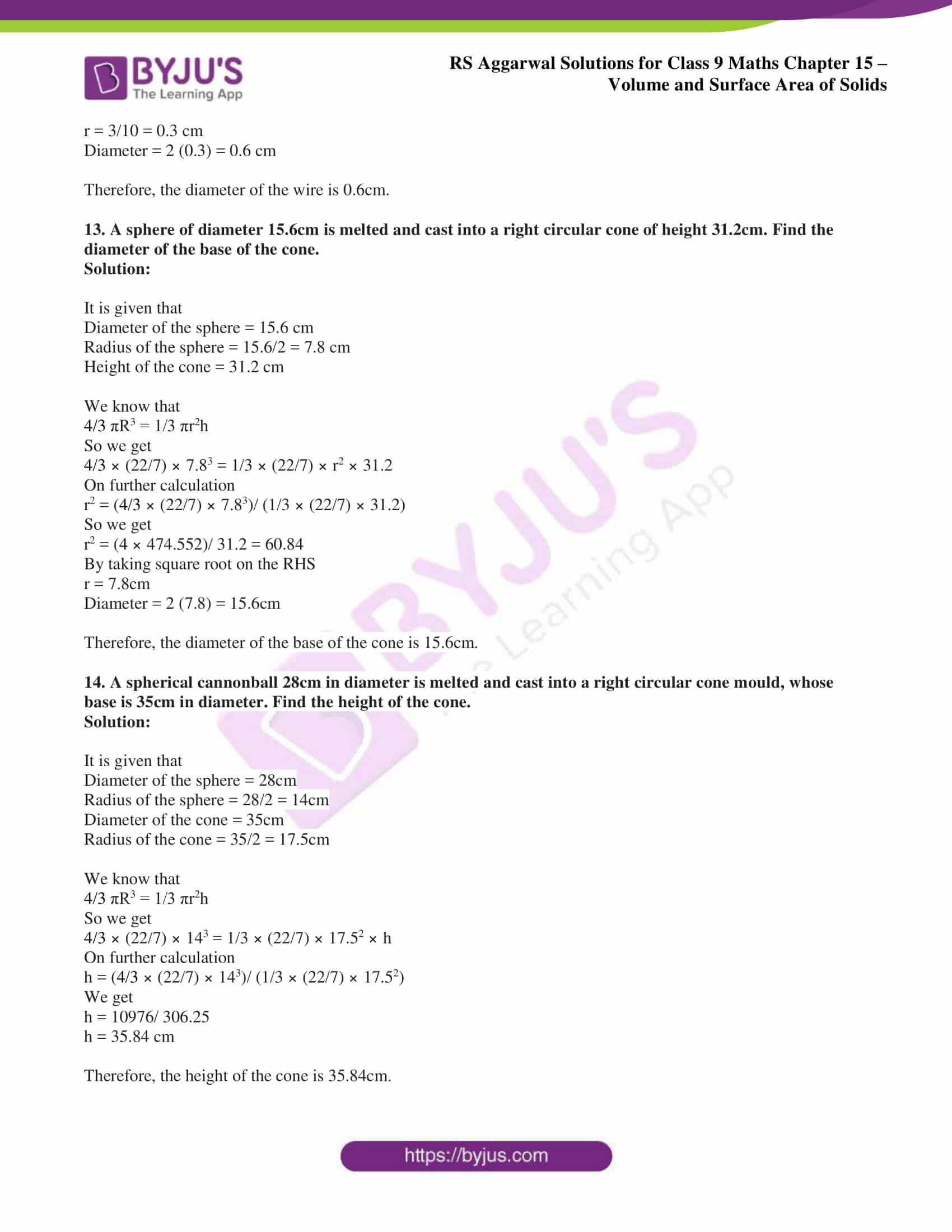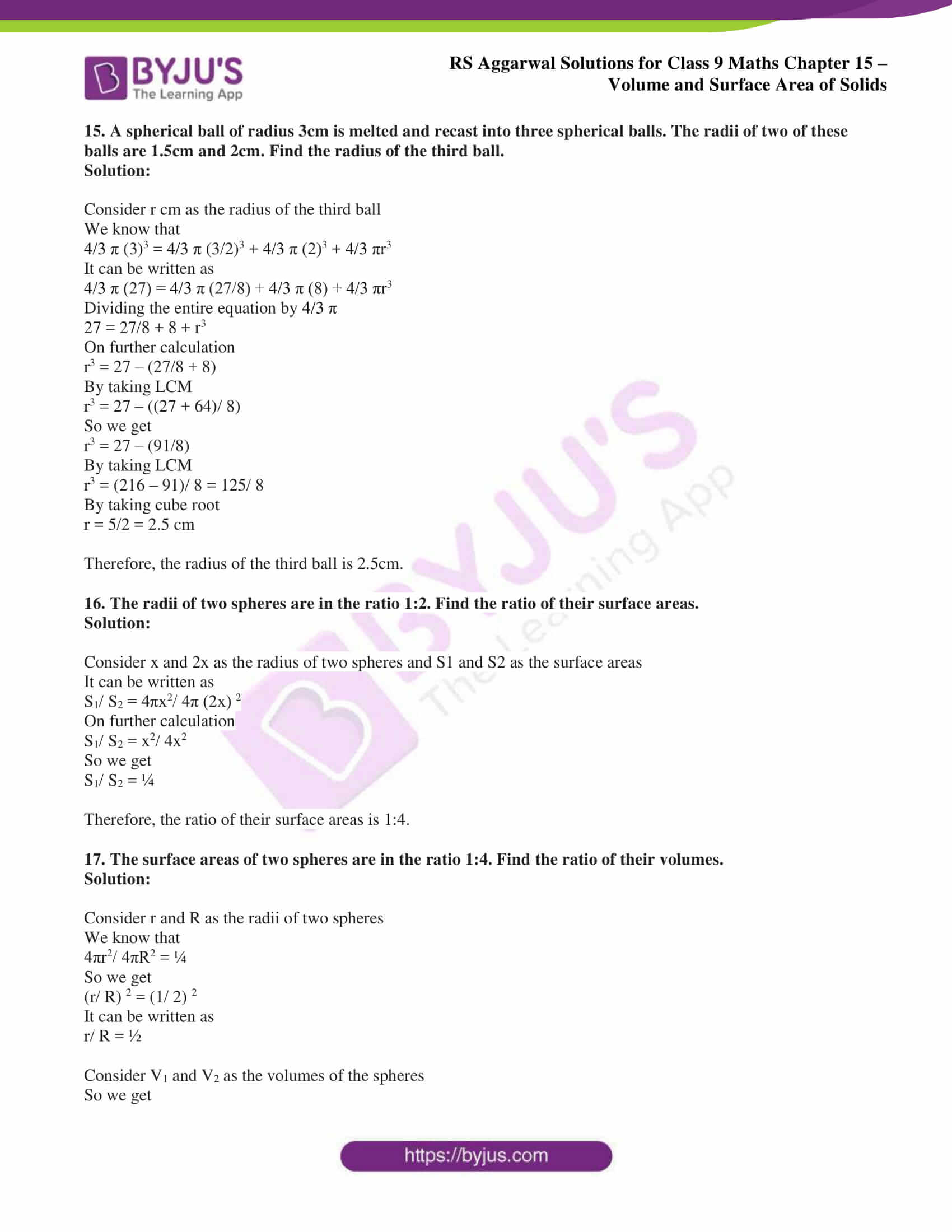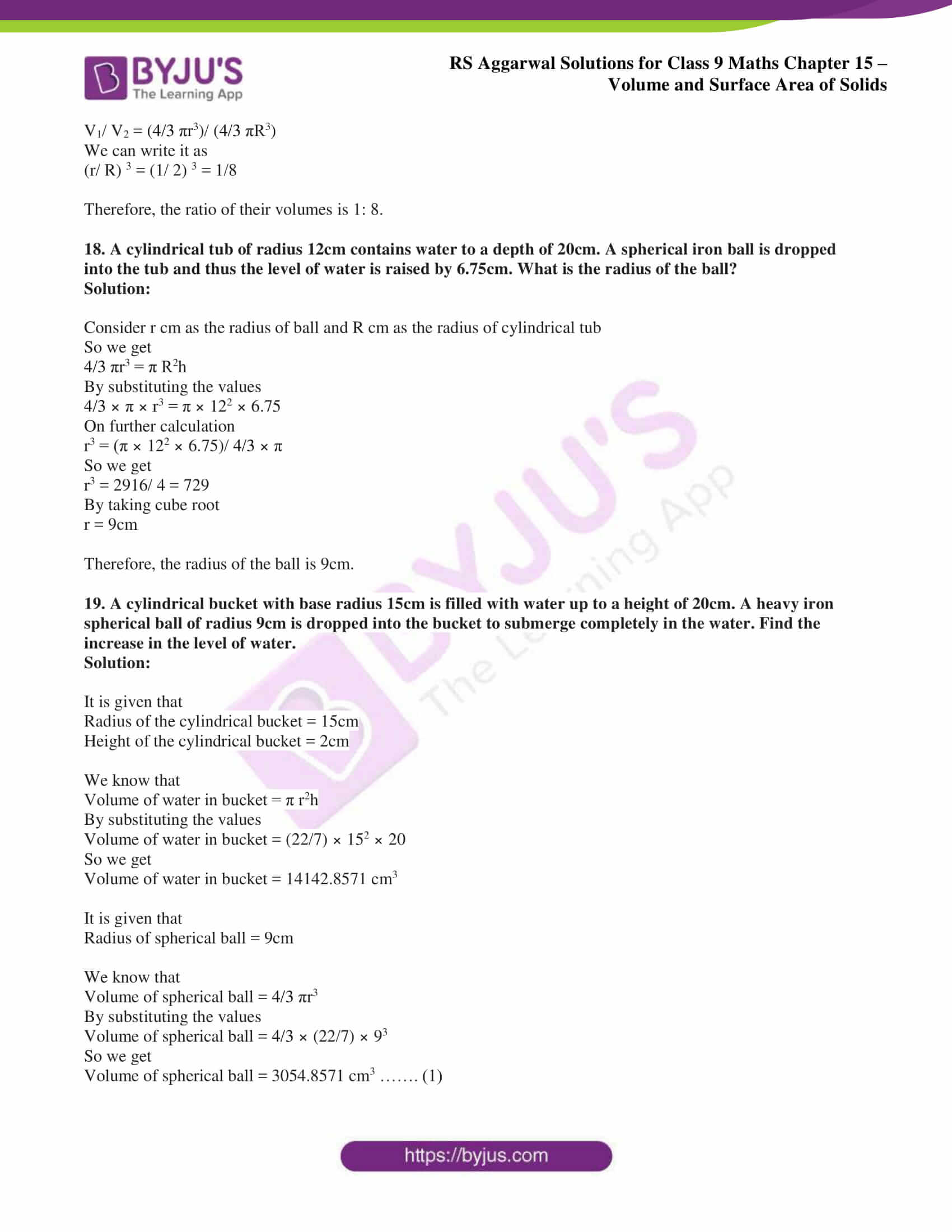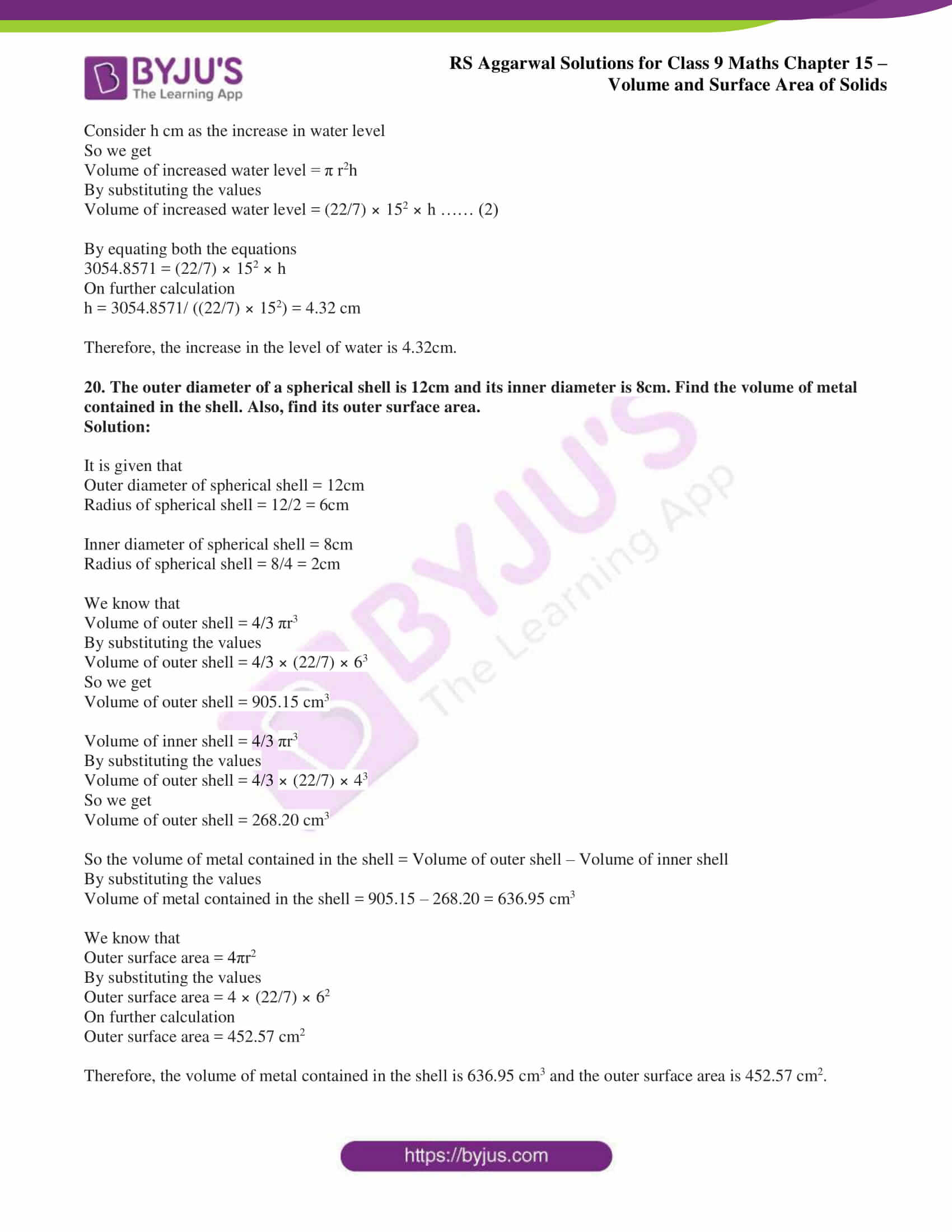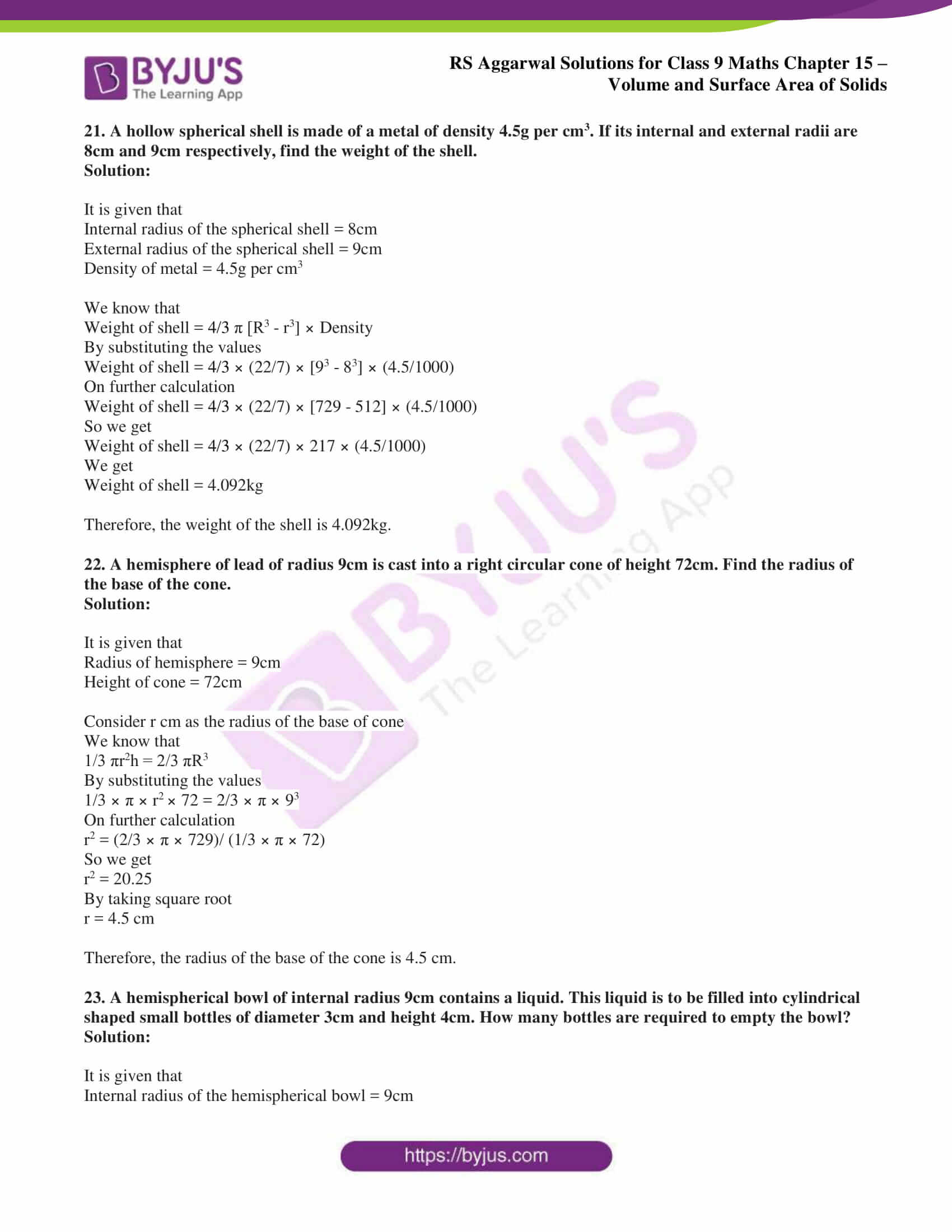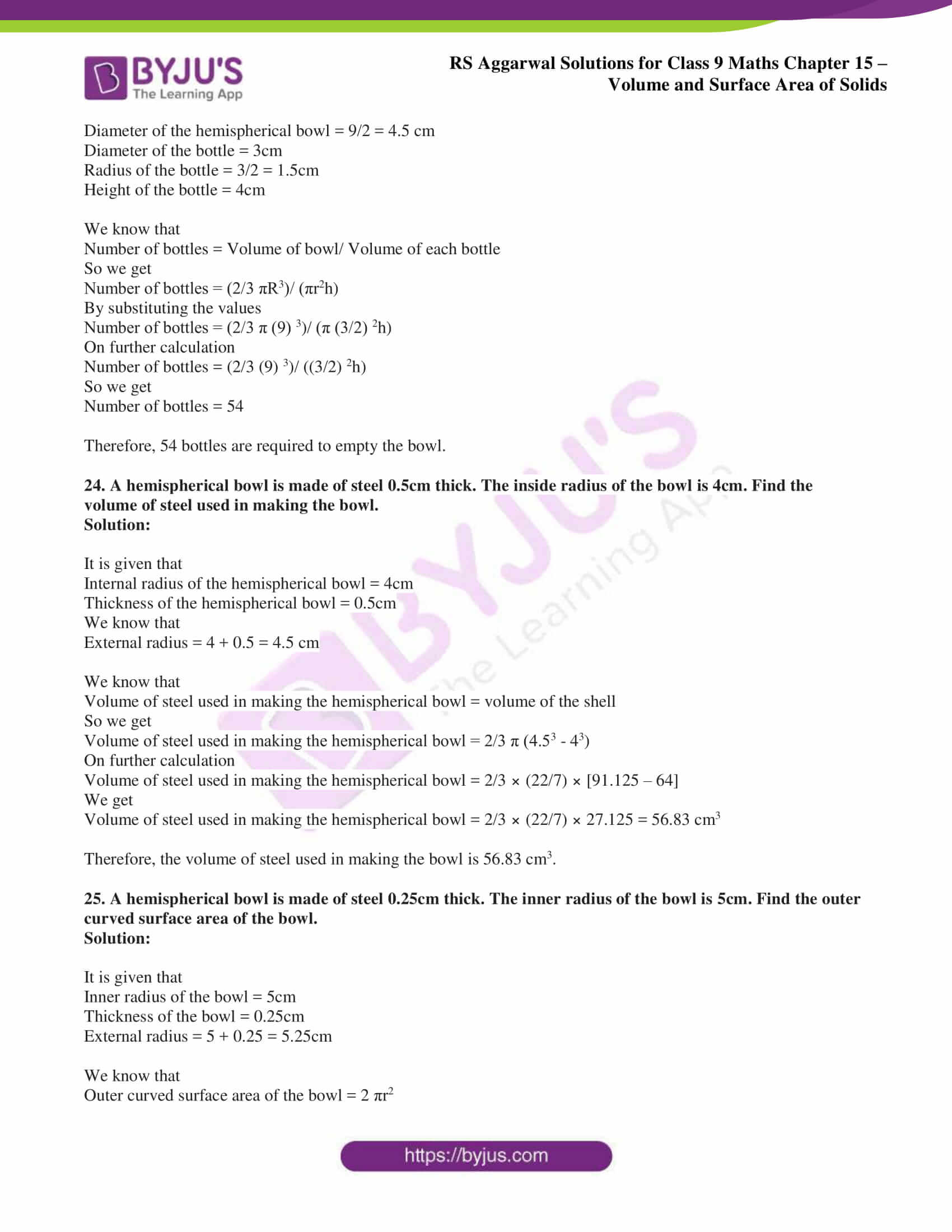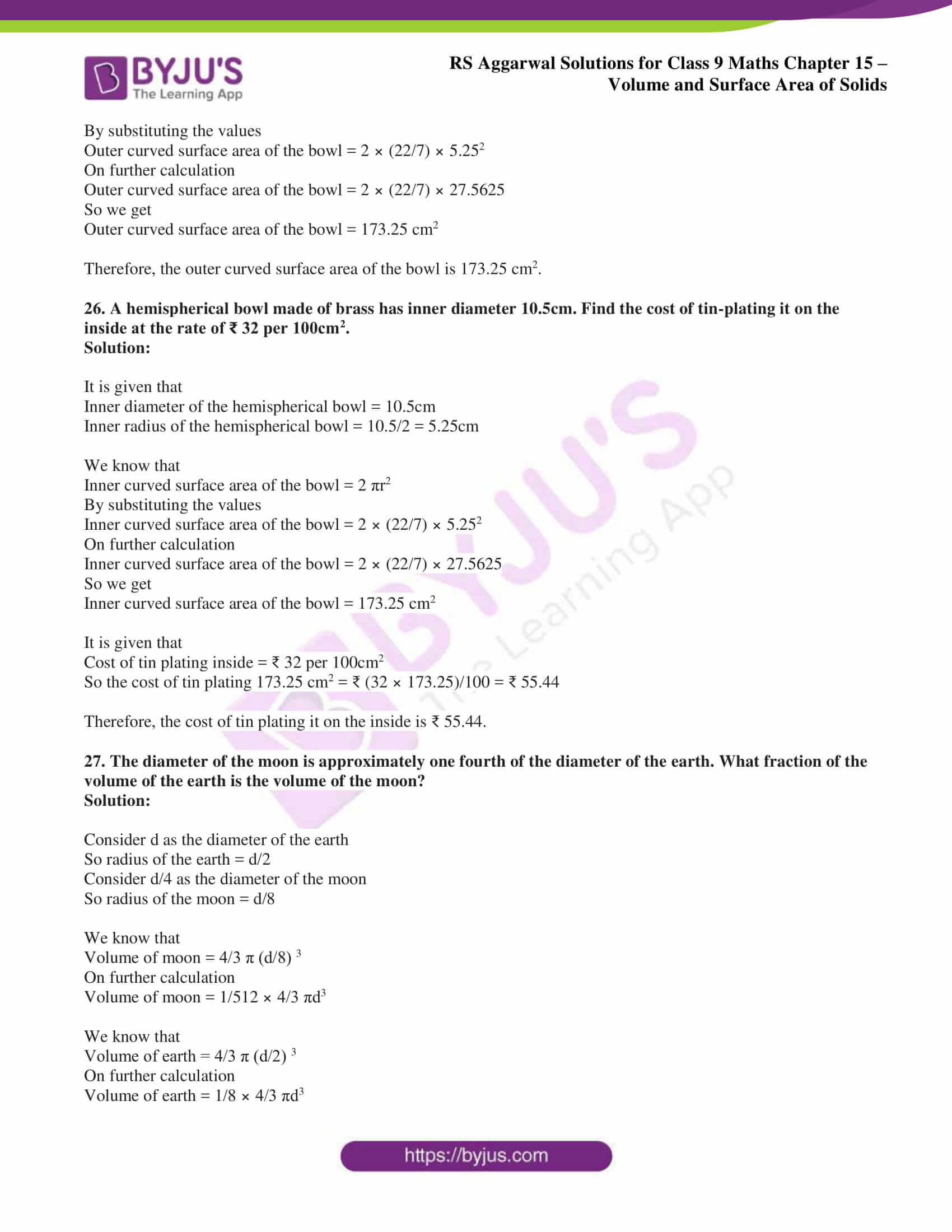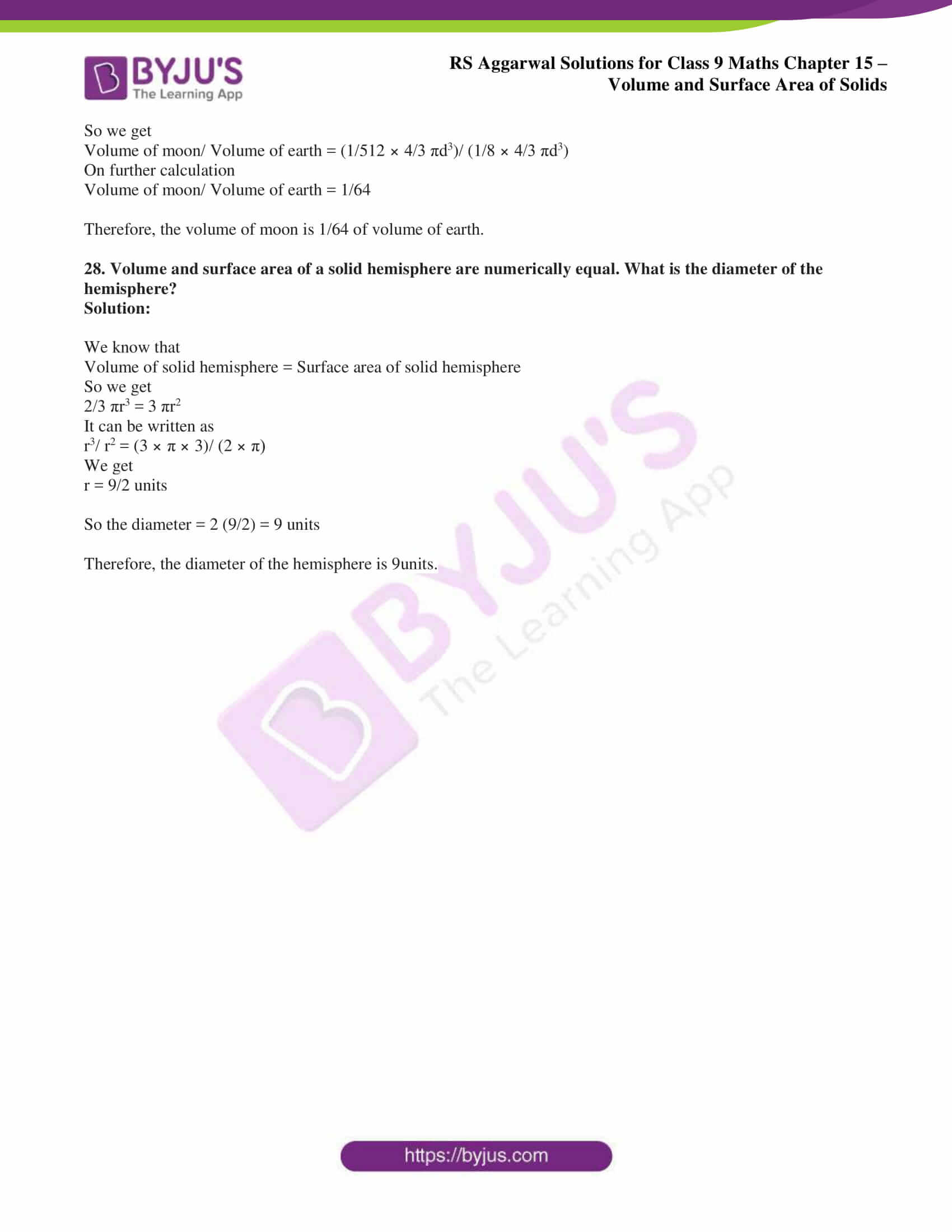## Access RS Aggarwal Solutions for Class 9 Chapter 15: Volumes and Surface Area of Solids Exercise 15D

1. Find the volume and surface area of a sphere whose radius is:

(i) 3.5 cm

(ii) 4.2 cm

(iii) 5m

Solution:

(i) It is given that

Radius of the sphere = 3.5cm

We know that

Volume of the sphere = 4/3 πr3

By substituting the values

Volume of the sphere = 4/3 × (22/7) × 3.53

So we get

Volume of the sphere = 179.67 cm3

We know that

Surface area of the sphere = 4 πr2

By substituting the values

Surface area of the sphere = 4 × (22/7) × 3.52

So we get

Surface area of the sphere = 154 cm2

(ii) It is given that

Radius of the sphere = 4.2cm

We know that

Volume of the sphere = 4/3 πr3

By substituting the values

Volume of the sphere = 4/3 × (22/7) × 4.23

So we get

Volume of the sphere = 310.464 cm3

We know that

Surface area of the sphere = 4 πr2

By substituting the values

Surface area of the sphere = 4 × (22/7) × 4.22

So we get

Surface area of the sphere = 221.76 cm2

(iii) It is given that

Radius of the sphere = 5cm

We know that

Volume of the sphere = 4/3 πr3

By substituting the values

Volume of the sphere = 4/3 × (22/7) × 53

So we get

Volume of the sphere = 523.81 m3

We know that

Surface area of the sphere = 4 πr2

By substituting the values

Surface area of the sphere = 4 × (22/7) × 52

So we get

Surface area of the sphere = 314.28 m2

2. The volume of a sphere is 38808 cm3. Find its radius and hence its surface area.

Solution:

We know that

Volume of the sphere = 4/3 πr3

By substituting the values

38808 = 4/3 × (22/7) × r3

On further calculation

r3 = (38808 × 3 × 7)/ 88

So we get

r3 = 9261

By taking cube root

r = 21cm

We know that

Surface area of the sphere = 4 πr2

By substituting the values

Surface area of the sphere = 4 × (22/7) × 212

So we get

Surface area of the sphere = 5544 cm2

Therefore, the radius of the sphere is 21cm and the surface area is 5544 cm2.

3. Find the surface area of a sphere whose volume is 606.375m3.

Solution:

We know that

Volume of the sphere = 4/3 πr3

By substituting the values

606.375 = 4/3 × (22/7) × r3

On further calculation

r3 = (606.375 × 3 × 7)/ 88

So we get

r3 = 144.703125

By taking cube root

r = 5.25m

We know that

Surface area of the sphere = 4 πr2

By substituting the values

Surface area of the sphere = 4 × (22/7) × 5.252

So we get

Surface area of the sphere = 346.5 m2

Therefore, the surface area of the sphere is 346.5 m2.

4. Find the volume of a sphere whose surface area is 154 cm2.

Solution:

We know that

Surface area of the sphere = 4 πr2

By substituting the values

4 πr2 = 154

On further calculation

4 × (22/7) × r2 = 154

So we get

r2 = (154 × 7)/ (4 × 22) = 49/4

By taking the square root

r = 7/2 cm

We know that

Volume of the sphere = 4/3 πr3

By substituting the values

Volume of the sphere = 4/3 × (22/7) × (7/2)3

So we get

Volume of the sphere = 179.67 cm3

Therefore, the volume of the sphere is 179.67 cm3.

5. The surface area of sphere is (576π) cm2. Find its volume.

Solution:

We know that

Surface area of the sphere = 4 πr2

By substituting the values

4 πr2 = 576π

On further calculation

r2 = 576/ 4 = 144

By taking square root

r = 12cm

We know that

Volume of the sphere = 4/3 πr3

By substituting the values

Volume of the sphere = 4/3 × π × (12)3

So we get

Volume of the sphere = 2304 π cm3

Therefore, the volume of the sphere is 2304 π cm3.

6. How many lead shots, each 3mm in diameter, can be made from a cuboid with dimensions (12cm × 11cm × 9cm)?

Solution:

It is given that

Diameter = 3mm = 0.3cm

We know that

Number of lead shots = volume of cuboid/ volume of 1 lead shot

By substituting the values

Number of lead shots = (12 × 11 × 9)/ (4/3 × (22/7) × (0.3/2)3)

On further calculation

Number of lead shots = (12 × 11 × 9)/ (4/3 × (22/7) × (0.027/8))

So we get

Number of lead shots = 84000

Therefore, the number of lead shots are 84000.

Solution:

It is given that

We know that

Number of lead balls = volume of sphere/ volume of one lead ball

So we get

Number of lead balls = (4/3 πR3)/ (4/3 πr3)

By substituting the values

Number of lead balls = (4/3 × (22/7) × 83)/ (4/3 × (22/7) × 13)

On further calculation

Number of lead balls = (4/3 × (22/7) × 512)/ (4/3 × (22/7) × 1)

We get

Number of lead balls = 512

8. A solid sphere of radius 3cm is melted and then cast into smaller spherical balls, each of diameter 6cm. Find the number of small balls thus obtained.

Solution:

It is given that

Diameter of spherical ball = 0.6cm

Radius of spherical ball = 0.6/2 = 0.3cm

We know that

Number of balls = Volume of sphere/ Volume of one small ball

So we get

Number of balls = (4/3 × (22/7) × 33)/ (4/3 × (22/7) × 0.33)

On further calculation

Number of balls = (4/3 × (22/7) × 27)/ (4/3 × (22/7) × 0.027)

We get

Number of balls = 1000

Therefore, 1000 balls are obtained from the solid sphere.

9. A metallic sphere of radius 10.5cm is melted and then recast into smaller cones, each of radius 3.5cm and height 3cm. How many cones are obtained?

Solution:

It is given that

Radius of the sphere = 10.5cm

Radius of smaller cone = 3.5cm

Height = 3cm

We know that

Number of cones = Volume of the sphere/ Volume of one small cone

So we get

Number of cones = (4/3 × (22/7) × 10.53)/ (1/3 × (22/7) × 3.52 × 3)

On further calculation

Number of cones = 4851/ 38.5 = 126

Therefore, 126 cones are obtained from the metallic sphere.

10. How many spheres 12cm in diameter can be made from a metallic cylinder of diameter 8cm and height 90cm?

Solution:

It is given that

Diameter of the sphere = 12cm

Radius of the sphere = 12/2 = 6cm

We know that

Volume of the sphere = 4/3 πr3

By substituting the values

Volume of the sphere = 4/3 × (22/7) × 63

So we get

Volume of the sphere = 905.142 cm3

It is given that

Diameter of the cylinder = 8cm

Radius of the cylinder = 8/2 = 4cm

Height of the cylinder = 90cm

We know that

Volume of the cylinder = πr2h

By substituting the values

Volume of the cylinder = (22/7) × 42 × 90

So we get

Volume of the cylinder = 4525.714 cm3

We know that

Number of spheres = Volume of cylinder/ Volume of sphere

By substituting the values

Number of spheres = 4525.714/ 905.142 = 5

Therefore, 5 spheres can be made from a metallic cylinder.

11. The diameter of a sphere is 6cm. It is melted and drawn into a wire of diameter 2mm. Find the length of the wire.

Solution:

It is given that

Diameter of the sphere = 6cm

Radius of the sphere = 6/2 = 3cm

Diameter of the wire = 2mm = 0.2 cm

Radius of the wire = 2/2 = 1mm = 0.1 cm

Consider h cm as the required length

So we get

πr2h = 4/3 πR3

By substituting the values

(22/7) × 0.12 × h = 4/3 × (22/7) × 33

On further calculation

h = (4/3 × (22/7) × 27)/ ((22/7) × 0.12)

So we get

h = 36/0.01 = 3600cm = 36m

Therefore, the length of the wire is 36m.

12. The diameter of a copper sphere is 18cm. It is melted and drawn into a long wire of uniform cross section. If the length of the wire is 108m, find its diameter.

Solution:

It is given that

Diameter of the sphere = 18cm

Radius of the sphere = 18/2 = 9cm

Length of the wire = 108m = 10800 cm

We know that

πr2h = 4/3 πr3

By substituting the values

(22/7) × r2 × 10800 = 4/3 × (22/7) × 93

On further calculation

r2 = (4/3 × (22/7) × 729)/ ((22/7) × 10800)

So we get

r2 = (4 × 243)/ 10800 = 9/100

By taking square root on the RHS

r = 3/10 = 0.3 cm

Diameter = 2 (0.3) = 0.6 cm

Therefore, the diameter of the wire is 0.6cm.

13. A sphere of diameter 15.6cm is melted and cast into a right circular cone of height 31.2cm. Find the diameter of the base of the cone.

Solution:

It is given that

Diameter of the sphere = 15.6 cm

Radius of the sphere = 15.6/2 = 7.8 cm

Height of the cone = 31.2 cm

We know that

4/3 πR3 = 1/3 πr2h

So we get

4/3 × (22/7) × 7.83 = 1/3 × (22/7) × r2 × 31.2

On further calculation

r2 = (4/3 × (22/7) × 7.83)/ (1/3 × (22/7) × 31.2)

So we get

r2 = (4 × 474.552)/ 31.2 = 60.84

By taking square root on the RHS

r = 7.8cm

Diameter = 2 (7.8) = 15.6cm

Therefore, the diameter of the base of the cone is 15.6cm.

14. A spherical cannonball 28cm in diameter is melted and cast into a right circular cone mould, whose base is 35cm in diameter. Find the height of the cone.

Solution:

It is given that

Diameter of the sphere = 28cm

Radius of the sphere = 28/2 = 14cm

Diameter of the cone = 35cm

Radius of the cone = 35/2 = 17.5cm

We know that

4/3 πR3 = 1/3 πr2h

So we get

4/3 × (22/7) × 143 = 1/3 × (22/7) × 17.52 × h

On further calculation

h = (4/3 × (22/7) × 143)/ (1/3 × (22/7) × 17.52)

We get

h = 10976/ 306.25

h = 35.84 cm

Therefore, the height of the cone is 35.84cm.

15. A spherical ball of radius 3cm is melted and recast into three spherical balls. The radii of two of these balls are 1.5cm and 2cm. Find the radius of the third ball.

Solution:

Consider r cm as the radius of the third ball

We know that

4/3 π (3)3 = 4/3 π (3/2)3 + 4/3 π (2)3 + 4/3 πr3

It can be written as

4/3 π (27) = 4/3 π (27/8) + 4/3 π (8) + 4/3 πr3

Dividing the entire equation by 4/3 π

27 = 27/8 + 8 + r3

On further calculation

r3 = 27 – (27/8 + 8)

By taking LCM

r3 = 27 – ((27 + 64)/ 8)

So we get

r3 = 27 – (91/8)

By taking LCM

r3 = (216 – 91)/ 8 = 125/ 8

By taking cube root

r = 5/2 = 2.5 cm

Therefore, the radius of the third ball is 2.5cm.

16. The radii of two spheres are in the ratio 1:2. Find the ratio of their surface areas.

Solution:

Consider x and 2x as the radius of two spheres and S1 and S2 as the surface areas

It can be written as

S1/ S2 = 4πx2/ 4π (2x) 2

On further calculation

S1/ S2 = x2/ 4x2

So we get

S1/ S2 = ¼

Therefore, the ratio of their surface areas is 1:4.

17. The surface areas of two spheres are in the ratio 1:4. Find the ratio of their volumes.

Solution:

Consider r and R as the radii of two spheres

We know that

4πr2/ 4πR2 = ¼

So we get

(r/ R) 2 = (1/ 2) 2

It can be written as

r/ R = ½

Consider V1 and V2 as the volumes of the spheres

So we get

V1/ V2 = (4/3 πr3)/ (4/3 πR3)

We can write it as

(r/ R) 3 = (1/ 2) 3 = 1/8

Therefore, the ratio of their volumes is 1: 8.

18. A cylindrical tub of radius 12cm contains water to a depth of 20cm. A spherical iron ball is dropped into the tub and thus the level of water is raised by 6.75cm. What is the radius of the ball?

Solution:

Consider r cm as the radius of ball and R cm as the radius of cylindrical tub

So we get

4/3 πr3 = π R2h

By substituting the values

4/3 × π × r3 = π × 122 × 6.75

On further calculation

r3 = (π × 122 × 6.75)/ 4/3 × π

So we get

r3 = 2916/ 4 = 729

By taking cube root

r = 9cm

Therefore, the radius of the ball is 9cm.

19. A cylindrical bucket with base radius 15cm is filled with water up to a height of 20cm. A heavy iron spherical ball of radius 9cm is dropped into the bucket to submerge completely in the water. Find the increase in the level of water.

Solution:

It is given that

Radius of the cylindrical bucket = 15cm

Height of the cylindrical bucket = 2cm

We know that

Volume of water in bucket = π r2h

By substituting the values

Volume of water in bucket = (22/7) × 152 × 20

So we get

Volume of water in bucket = 14142.8571 cm3

It is given that

Radius of spherical ball = 9cm

We know that

Volume of spherical ball = 4/3 πr3

By substituting the values

Volume of spherical ball = 4/3 × (22/7) × 93

So we get

Volume of spherical ball = 3054.8571 cm3 ……. (1)

Consider h cm as the increase in water level

So we get

Volume of increased water level = π r2h

By substituting the values

Volume of increased water level = (22/7) × 152 × h …… (2)

By equating both the equations

3054.8571 = (22/7) × 152 × h

On further calculation

h = 3054.8571/ ((22/7) × 152) = 4.32 cm

Therefore, the increase in the level of water is 4.32cm.

20. The outer diameter of a spherical shell is 12cm and its inner diameter is 8cm. Find the volume of metal contained in the shell. Also, find its outer surface area.

Solution:

It is given that

Outer diameter of spherical shell = 12cm

Radius of spherical shell = 12/2 = 6cm

Inner diameter of spherical shell = 8cm

Radius of spherical shell = 8/4 = 2cm

We know that

Volume of outer shell = 4/3 πr3

By substituting the values

Volume of outer shell = 4/3 × (22/7) × 63

So we get

Volume of outer shell = 905.15 cm3

Volume of inner shell = 4/3 πr3

By substituting the values

Volume of outer shell = 4/3 × (22/7) × 43

So we get

Volume of outer shell = 268.20 cm3

So the volume of metal contained in the shell = Volume of outer shell – Volume of inner shell

By substituting the values

Volume of metal contained in the shell = 905.15 – 268.20 = 636.95 cm3

We know that

Outer surface area = 4πr2

By substituting the values

Outer surface area = 4 × (22/7) × 62

On further calculation

Outer surface area = 452.57 cm2

Therefore, the volume of metal contained in the shell is 636.95 cm3 and the outer surface area is 452.57 cm2.

21. A hollow spherical shell is made of a metal of density 4.5g per cm3. If its internal and external radii are 8cm and 9cm respectively, find the weight of the shell.

Solution:

It is given that

Internal radius of the spherical shell = 8cm

External radius of the spherical shell = 9cm

Density of metal = 4.5g per cm3

We know that

Weight of shell = 4/3 π [R3 – r3] × Density

By substituting the values

Weight of shell = 4/3 × (22/7) × [93 – 83] × (4.5/1000)

On further calculation

Weight of shell = 4/3 × (22/7) × [729 – 512] × (4.5/1000)

So we get

Weight of shell = 4/3 × (22/7) × 217 × (4.5/1000)

We get

Weight of shell = 4.092kg

Therefore, the weight of the shell is 4.092kg.

22. A hemisphere of lead of radius 9cm is cast into a right circular cone of height 72cm. Find the radius of the base of the cone.

Solution:

It is given that

Height of cone = 72cm

Consider r cm as the radius of the base of cone

We know that

1/3 πr2h = 2/3 πR3

By substituting the values

1/3 × π × r2 × 72 = 2/3 × π × 93

On further calculation

r2 = (2/3 × π × 729)/ (1/3 × π × 72)

So we get

r2 = 20.25

By taking square root

r = 4.5 cm

Therefore, the radius of the base of the cone is 4.5 cm.

23. A hemispherical bowl of internal radius 9cm contains a liquid. This liquid is to be filled into cylindrical shaped small bottles of diameter 3cm and height 4cm. How many bottles are required to empty the bowl?

Solution:

It is given that

Internal radius of the hemispherical bowl = 9cm

Diameter of the hemispherical bowl = 9/2 = 4.5 cm

Diameter of the bottle = 3cm

Radius of the bottle = 3/2 = 1.5cm

Height of the bottle = 4cm

We know that

Number of bottles = Volume of bowl/ Volume of each bottle

So we get

Number of bottles = (2/3 πR3)/ (πr2h)

By substituting the values

Number of bottles = (2/3 π (9) 3)/ (π (3/2) 2h)

On further calculation

Number of bottles = (2/3 (9) 3)/ ((3/2) 2h)

So we get

Number of bottles = 54

Therefore, 54 bottles are required to empty the bowl.

24. A hemispherical bowl is made of steel 0.5cm thick. The inside radius of the bowl is 4cm. Find the volume of steel used in making the bowl.

Solution:

It is given that

Internal radius of the hemispherical bowl = 4cm

Thickness of the hemispherical bowl = 0.5cm

We know that

External radius = 4 + 0.5 = 4.5 cm

We know that

Volume of steel used in making the hemispherical bowl = volume of the shell

So we get

Volume of steel used in making the hemispherical bowl = 2/3 π (4.53 – 43)

On further calculation

Volume of steel used in making the hemispherical bowl = 2/3 × (22/7) × [91.125 – 64]

We get

Volume of steel used in making the hemispherical bowl = 2/3 × (22/7) × 27.125 = 56.83 cm3

Therefore, the volume of steel used in making the bowl is 56.83 cm3.

25. A hemispherical bowl is made of steel 0.25cm thick. The inner radius of the bowl is 5cm. Find the outer curved surface area of the bowl.

Solution:

It is given that

Inner radius of the bowl = 5cm

Thickness of the bowl = 0.25cm

External radius = 5 + 0.25 = 5.25cm

We know that

Outer curved surface area of the bowl = 2 πr2

By substituting the values

Outer curved surface area of the bowl = 2 × (22/7) × 5.252

On further calculation

Outer curved surface area of the bowl = 2 × (22/7) × 27.5625

So we get

Outer curved surface area of the bowl = 173.25 cm2

Therefore, the outer curved surface area of the bowl is 173.25 cm2.

26. A hemispherical bowl made of brass has inner diameter 10.5cm. Find the cost of tin-plating it on the inside at the rate of ₹ 32 per 100cm2.

Solution:

It is given that

Inner diameter of the hemispherical bowl = 10.5cm

Inner radius of the hemispherical bowl = 10.5/2 = 5.25cm

We know that

Inner curved surface area of the bowl = 2 πr2

By substituting the values

Inner curved surface area of the bowl = 2 × (22/7) × 5.252

On further calculation

Inner curved surface area of the bowl = 2 × (22/7) × 27.5625

So we get

Inner curved surface area of the bowl = 173.25 cm2

It is given that

Cost of tin plating inside = ₹ 32 per 100cm2

So the cost of tin plating 173.25 cm2 = ₹ (32 × 173.25)/100 = ₹ 55.44

Therefore, the cost of tin plating it on the inside is ₹ 55.44.

27. The diameter of the moon is approximately one fourth of the diameter of the earth. What fraction of the volume of the earth is the volume of the moon?

Solution:

Consider d as the diameter of the earth

So radius of the earth = d/2

Consider d/4 as the diameter of the moon

So radius of the moon = d/8

We know that

Volume of moon = 4/3 π (d/8) 3

On further calculation

Volume of moon = 1/512 × 4/3 πd3

We know that

Volume of earth = 4/3 π (d/2) 3

On further calculation

Volume of earth = 1/8 × 4/3 πd3

So we get

Volume of moon/ Volume of earth = (1/512 × 4/3 πd3)/ (1/8 × 4/3 πd3)

On further calculation

Volume of moon/ Volume of earth = 1/64

Therefore, the volume of moon is 1/64 of volume of earth.

28. Volume and surface area of a solid hemisphere are numerically equal. What is the diameter of the hemisphere?

Solution:

We know that

Volume of solid hemisphere = Surface area of solid hemisphere

So we get

2/3 πr3 = 3 πr2

It can be written as

r3/ r2 = (3 × π × 3)/ (2 × π)

We get

r = 9/2 units

So the diameter = 2 (9/2) = 9 units

Therefore, the diameter of the hemisphere is 9units.

### Access other exercise solutions of Class 9 Maths Chapter 15: Volume and Surface Area of Solids

Exercise 15A Solutions 28 Questions

Exercise 15B Solutions 33 Questions

Exercise 15C Solutions 21 Questions

### RS Aggarwal Solutions Class 9 Maths Chapter 15 – Volume and Surface Area of Solids Exercise 15D

RS Aggarwal Solutions Class 9 Maths Chapter 15 Volume and Surface Area of Solids Exercise 15D covers concepts like sphere, spherical shell and hemisphere along with basic formulas used in solving problems based on them.

### Key features of RS Aggarwal Solutions for Class 9 Maths Chapter 15: Volume and Surface Area of Solids Exercise 15D

• The students can self assess their learning abilities and work on areas which require more importance based on weightage.
• Solving problems from RS Aggarwal textbook help students to appear for exams with confidence.
• The examples and huge number of exercise wise problems in each chapter help students improve their speed in solving them.
• The important formulas which are required to solve the problems can be remembered easily.